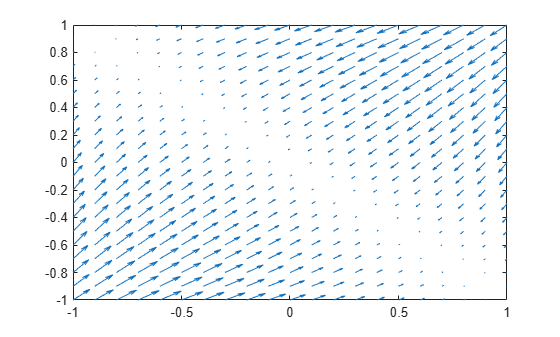Main Content

# gradient

Gradient vector of scalar function

## Syntax

``gradient(f,v)``

## Description

example

````gradient(f,v)` finds the gradient vector of the scalar function `f` with respect to vector `v` in Cartesian coordinates.If you do not specify `v`, then `gradient(f)` finds the gradient vector of the scalar function `f` with respect to a vector constructed from all symbolic variables found in `f`. The order of variables in this vector is defined by `symvar`.```

## Examples

### Find Gradient of Function

The gradient of a function `f` with respect to the vector `v` is the vector of the first partial derivatives of `f` with respect to each element of `v`.

Find the gradient vector of `f(x, y, z)` with respect to vector `[x, y, z]`. The gradient is a vector with these components.

```syms x y z f = 2*y*z*sin(x) + 3*x*sin(z)*cos(y); gradient(f, [x, y, z])```
```ans = 3*cos(y)*sin(z) + 2*y*z*cos(x) 2*z*sin(x) - 3*x*sin(y)*sin(z) 2*y*sin(x) + 3*x*cos(y)*cos(z)```

### Plot Gradient of Function

Find the gradient of a function `f(x,y)`, and plot it as a quiver (velocity) plot.

Find the gradient vector of `f(x,y)` with respect to vector `[x,y]`. The gradient is vector `g` with these components.

```syms x y f = -(sin(x) + sin(y))^2; g = gradient(f,[x,y])```
```g =  $\left(\begin{array}{c}-2 \mathrm{cos}\left(x\right) \left(\mathrm{sin}\left(x\right)+\mathrm{sin}\left(y\right)\right)\\ -2 \mathrm{cos}\left(y\right) \left(\mathrm{sin}\left(x\right)+\mathrm{sin}\left(y\right)\right)\end{array}\right)$```

Now plot the vector field defined by these components. MATLAB® provides the `quiver` plotting function for this task. The function does not accept symbolic arguments. First, replace symbolic variables in expressions for components of `g` with numeric values. Then use `quiver`.

```[X, Y] = meshgrid(-1:.1:1,-1:.1:1); G1 = subs(g(1),[x y],{X,Y}); G2 = subs(g(2),[x y],{X,Y}); quiver(X,Y,G1,G2)```## Input Arguments

collapse all

Scalar function, specified as symbolic expression or symbolic function.

Vector with respect to which you find gradient vector, specified as a symbolic vector. By default, `v` is a vector constructed from all symbolic variables found in `f`. The order of variables in this vector is defined by `symvar`.

If `v` is a scalar, ```gradient(f,v) = diff(f,v)```. If `v` is an empty symbolic object, such as `sym([])`, then `gradient` returns an empty symbolic object.

## More About

collapse all

### Gradient Vector

The gradient vector of f(x) with respect to the vector x is the vector of the first partial derivatives of `f`.

`$\nabla f=\left(\frac{\partial f}{\partial {x}_{1}},\frac{\partial f}{\partial {x}_{2}},\dots ,\frac{\partial f}{\partial {x}_{n}}\right)$`

## See Also

Introduced in R2011b

## Support

#### Mathematical Modeling with Symbolic Math Toolbox

Get examples and videos